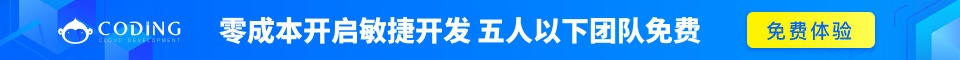# Python 循环嵌套

Python 语言允许在一个循环体里面嵌入另一个循环。

Python for 循环嵌套语法：

```for iterating_var in sequence:
for iterating_var in sequence:
statements(s)
statements(s)```

Python while 循环嵌套语法：

```while expression:
while expression:
statement(s)
statement(s)```

1. 从第一个列表中每次取出一个，从第二个列表中也每次取出一个，组合成一个新的列表，新列表中包含所有组合

```List1 = ['zi', 'qiang', 'xue', 'tang']
List2 = [1, 2]

new_list = []

for m in List1:
for n in List2:
new_list.append([m, n])

print new_list```

`[['zi', 1], ['zi', 2], ['qiang', 1], ['qiang', 2], ['xue', 1], ['xue', 2], ['tang', 1], ['tang', 2]]`

2. 从一个列表中每次取出两个，找出所有组合方式

```List = [1, 2, 3, 4, 5]
length = len(List)

for x in range(0, length-1):
for y in range(x+1, length):
print List[x], List[y]```

```1 2
1 3
1 4
1 5
2 3
2 4
2 5
3 4
3 5
4 5```

3. 以下实例使用了嵌套循环输出2~20之间的素数：

3.1 用 for 来实现

```# -*- coding: utf-8 -*-

n = 20

for i in range(1, n):
for j in range(2, int(i**0.5)+1):
if i % j == 0:
break
else:
print i, '是素数'```

3.2 用 while 来实现

```#!/usr/bin/python
# -*- coding: utf-8 -*-

i = 2
while(i < 20):
j = 2
while(j <= (i/j)):
if not(i%j):  #  或者写 if i % j == 0，如果整除的意思
break
j = j + 1
if (j > i/j):
print i, " 是素数"
i = i + 1

print "Good bye!"```

```2 是素数
3 是素数
5 是素数
7 是素数
11 是素数
13 是素数
17 是素数
Good bye!```